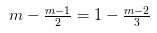# Solve the following linear equations: m - m-1/2 = 1 - m - 2/3

Solve the following linear equations: